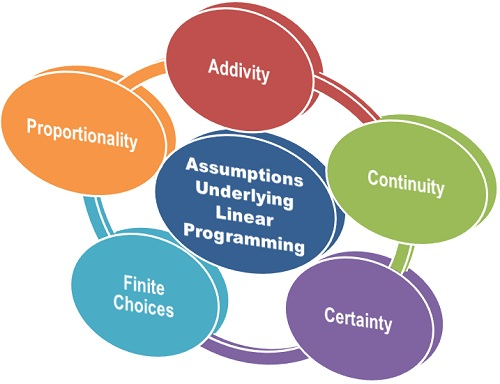# Linear Programming Problem

The Linear Programming problem is formulated to determine the optimum solution by selecting the best alternative from the set of feasible alternatives available to the decision maker.

Assumptions of Linear programming

There are several assumptions on which the linear programming works, these are:1. Proportionality: The basic assumption underlying the linear programming is that any change in the constraint inequalities will have the proportional change in the objective function. This means, if product contributes Rs 20 towards the profit, then the total contribution would be equal to 20×1, where x1 is the number of units of the product.

For example, if there are 5 units of the product, then the contribution would be Rs 100 and in the case of 10 units, it would be Rs 200. Thus, if the output (sales) is doubled, the profit would also be doubled.

1. Additivity: The assumption of additivity asserts that the total profit of the objective function is determined by the sum of profit contributed by each product separately. Similarly, the total amount of resources used is determined by the sum of resources used by each product separately. This implies, there is no interaction between the decision variables.
2. Continuity: Another assumption of linear programming is that the decision variables are continuous. This means a combination of outputs can be used with the fractional values along with the integer values.

For example, If 52/3 units of product A and 101/3 units of product B to be produced in a week. In this case, the fractional amount of production will be taken as a work-in-progress and the remaining production part is taken in the following week. Therefore, a production of 17 units of product A and 31 units of product B over a three-week period implies 52/3 units of product A and 101/3 units of product B per week.

1. Certainty: Another underlying assumption of linear programming is a certainty, i.e. the parameters of objective function coefficients and the coefficients of constraint inequalities is known with certainty. Such as profit per unit of product, availability of material and labor per unit, requirement of material and labor per unit are known and is given in the linear programming problem.
2. Finite Choices: This assumption implies that the decision maker has certain choices, and the decision variables assume non-negative values. The non-negative assumption is true in the sense, the output in the production problem can not be negative. Thus, this assumption is considered feasible.

Thus, while solving for the linear programming problem, these assumptions should be kept in mind such that the best alternative is chosen.

## One thought on “Linear Programming Problem”

error: Content is protected !!